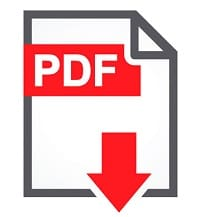# Enable Validation in List Definition from Visual Studio 2010 in SharePoint 2010

This SharePoint tutorial, we discuss how we enable the validation in list definition using visual studio 2010.

1. First, we need to create the List definition using visual studio 2010.

2. Now we add the two-column Start date and End date in element.xml and Schema.xml.

3. Now See the following code for adding the validation in End Date column and add that code under Element.xml and Schema.xml. We also give the message if the condition is false. See in code

<Field ID=”{E01F3A97-3526-4073-BFE6-FB7A543488DF}” Name=”Start_Date” DisplayName=”Start Date” Type=”DateTime” Required=”TRUE”></Field>

<Field ID=”{F56FFE7E-9698-470E-9882-4F8CE28ADBA5}” Name=”End_Date” DisplayName=”End Date” Type=”DateTime” Required=”TRUE”>
<Validation Message=”End Date must be larger than or equal to Start Date”>=[End_Date]>=[Start_Date]</Validation>
</Field>

4. Now we save and deploy the solution.

5. Now we go to our list definition and add the item.

6. Now if the condition is true then the item is added but if the condition is false then the message is showing. See fig.Enable Validation in List Definition from Visual Studio 2010 in SharePoint 2010

You may like following SharePoint tutorials: